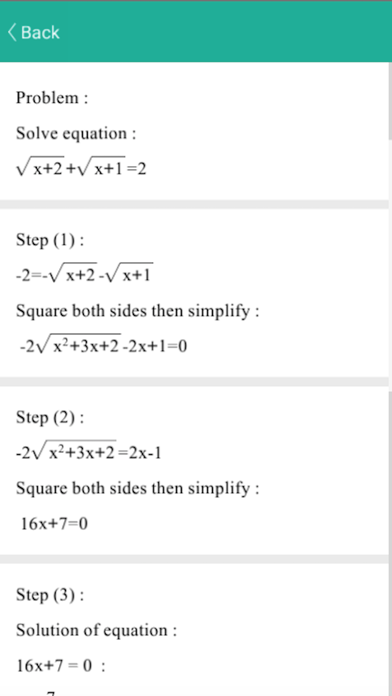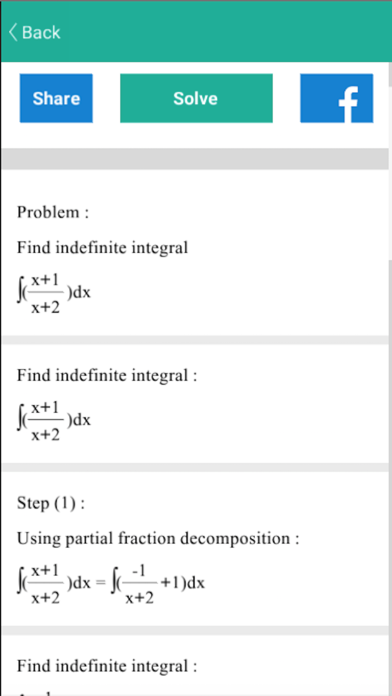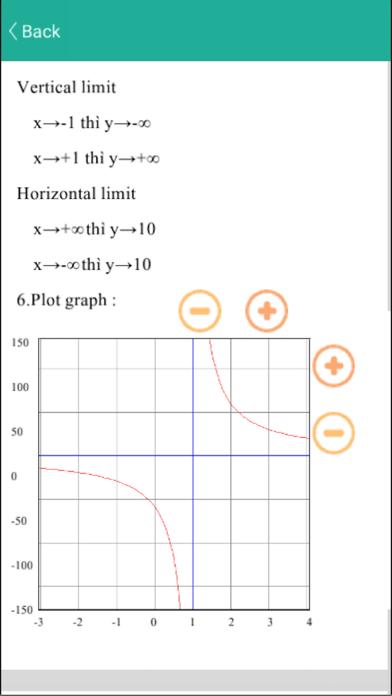## Maths Solver - Lumos Educational App Store4.21
Price -Free
\$0

#### DESCRIPTION:

Math solver is an easy to use application for all students it can solve step-by-step for most high school mathematic problems like: - Finding limits - Graphing the functions - Polynomial equations: quaratic, cubic functions. - Rational and irrational equations - Solving Trigonometric equations - Solving System fo equations: can solve linear and non-linear equations, up to 5 variables - Solving inequality. - Finding derivatives - Factorization. - Indefinite and definite integrations - Matrix operations: addition, substraction, multiplication, inverse. You can hit “share” to send lat

#### OVERVIEW:

Maths Solver is a free educational mobile app By Canh Pham Khac.It helps students practice the following standards .

This page not only allows students and teachers download Maths Solver but also find engaging Sample Questions, Videos, Pins, Worksheets, Books related to the following topics.

Developer: Canh Pham Khac

Software Version: 2.3

Category: Education

Release Date: 2017-03-16T16:32:56Z#### Are you the Developer?EdSearch WebSearch